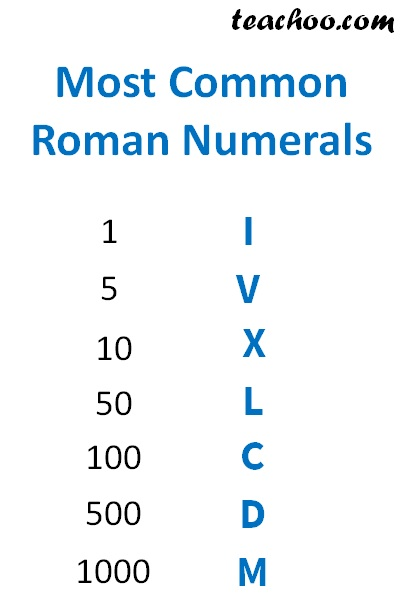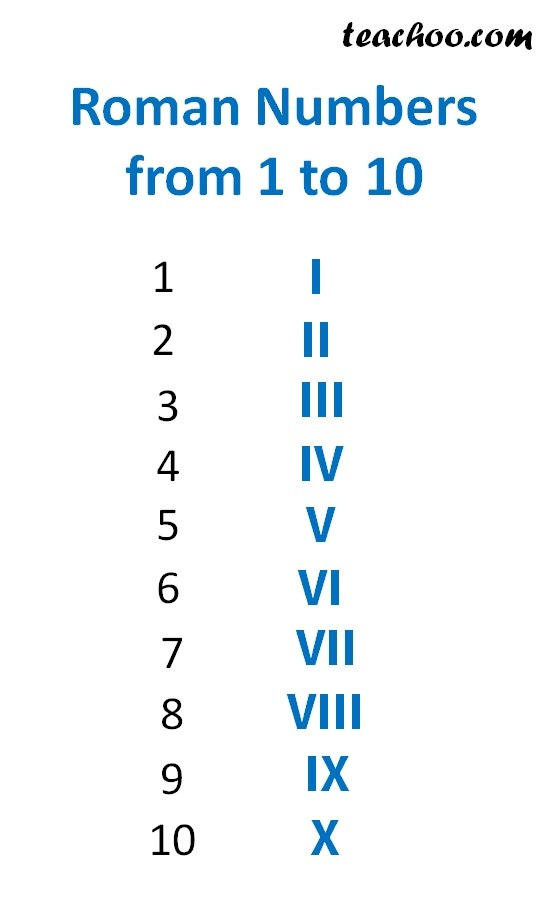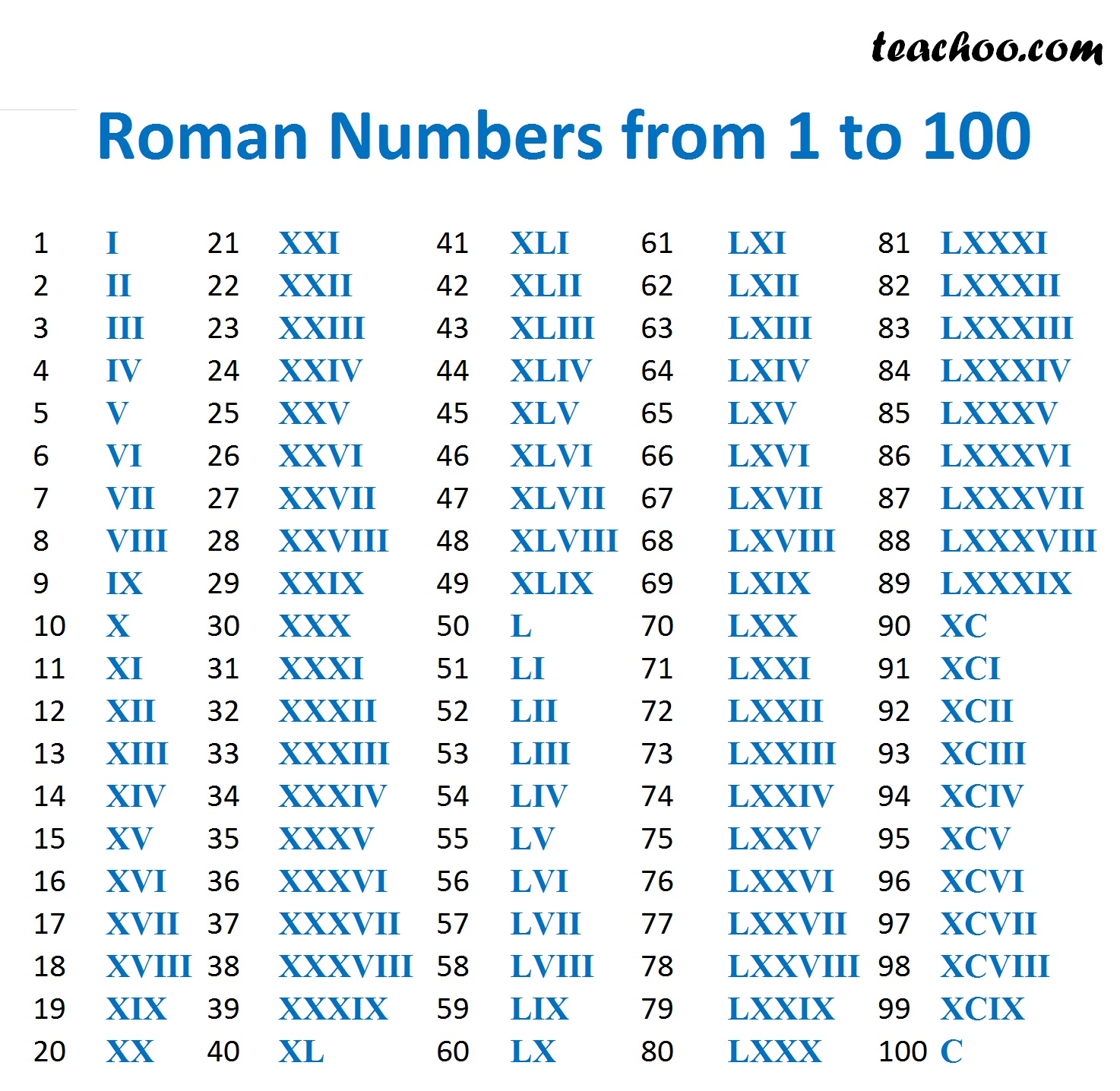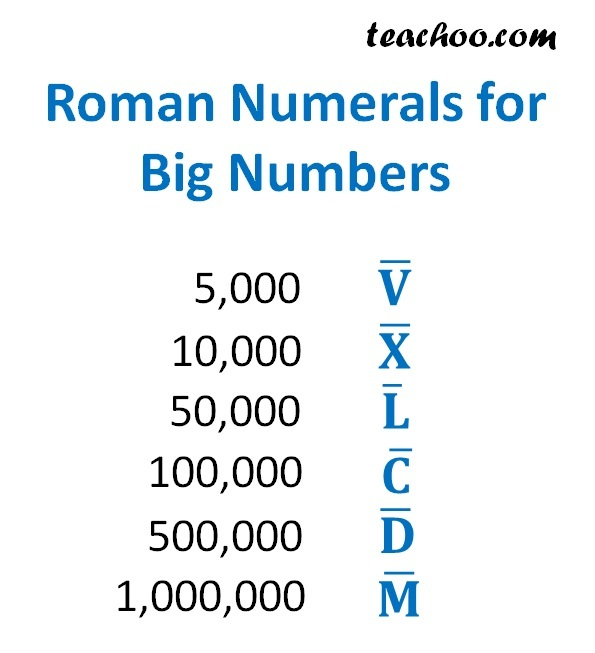Roman numerals

Chapter 1 Class 6 Knowing our Numbers
Concept wise

Have you ever seenOrThese are Roman Numerals

Like we write

1, 2, 3, 4, ………

Romans write like

I, II, III, IV, ……..

These are called Roman Numbers.

Here is another example of using Roman numerals in a clock## Most common roman numeralsAll other roman numerals are  made from this

## Rules for Forming Roman Numbers

Let's keep our table here for reference

 Numbers Roman Numbers 1 I 5 V 10 X 50 L 100 C 500 D 1000 M

### Rule 1

If symbol is repeated, it’s value is added

Example

II = I + I = 1 + 1 = 2

XXX = X + X + X = 10 + 10 + 10 = 30

### Rule 2

No symbol is repeated more than 3 times

III is allowed

IIII is not allowed

Note : V, L, D are never repeated.

### Rule 3

If smaller value symbol is written to right of greater value symbol, its value gets added .

VI = V + I = 5 + 1 = 6

XII = X + I + I = 10 + 1 + 1 = 12

LXV = L + X + V = 50 + 10 + 5 = 65

### Rule 4

If the symbol of smaller value is written to the   left of greater value, its value gets subtracted

IV = V − I = 5 − 1 = 4

IX = X − I = 10 − 1 = 9

XL = L − X = 50 − 10 = 40

XC = C − X = 100 − 10 = 90

Let's take some examples

Lets take some examples

#### Write 16 as roman numeral

16 = 10 + 5 + 1

= X + V + I

= XVI

#### Write 26 as roman numeral

20 = 20 + 6

= 10 + 10 + 6

= 10 + 10 + 5 + 1

= X + X + V + I

= XXVI

#### Write 34 as roman numeral

34 = 30 + 4

= 10 + 10 + 10 + 4

= 10 + 10 + 10 + (5 - 1)

= X + X + X + (V - I)

= X + X + X + IV

= XXXIV

#### Write 59 as roman numeral

50 = 50 + 9

= 50 + (10 – 1)

= L + (X – I)

= L + IX

= LIX

#### Write 183 as roman numeral

183 = 100 + 50 + 33

= 100 + 50 + 10 + 10 + 10 + 3

= C + L + X + X + X + III

= CLXXXIII

#### Write 556 as roman numeral

556 = 500 + 50 + 6

= 500 + 50 + (5 + 1)

= D + L + V + I

= DLVI

#### Write 1589 as roman numeral

1589 = 1000 + 500 + 50 + 30 + 9

= 1000 + 500 + 50 + 10  + 10  + 10 + (10 – 1)

= M + D + L + X + X + X + (X – I)

= M + D + L + X + X + X + IX

= MDLXXXIX

## Roman Numbers from 1 to 10## Roman numbers from 1 to 100## Roman Numerals for Big Numbers